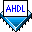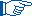## Boolean Equation Rules

 See AlsoThe following rules apply to Boolean equations:

• Multiple assignments to a variable are logically ORed (`#`), except when the default for the variable is `VCC`, in which case the assignments are logically ANDed (`&`).

• If the number of nodes on the left of the Boolean equation equals the number of nodes on the right, a one-to-one correspondence exists.

• If a single node, `GND`, or `VCC` on the right of an equation is assigned to a group, the node or constant is duplicated to match the size of the group. Example

• If both the left and right of the equation are groups of the same size, each member on the right is assigned to the member on the left that corresponds in position. ExampleWhen you add two groups together on the right of a Boolean equation with the `+` operator, you can place a `0` on the left of each group to sign-extend the width of the group. This method provides an extra bit of information to the group on the left of the equation that can be used as a carry-out signal. Example
• If the left and right of an equation have groups of different sizes, the number of bits in the group on the left must be evenly divisible by the number of bits in the group on the right. The bits on the left of the equation are mapped to the right of the equation, in order. Example

• A group of nodes or numbers cannot be assigned to a single node.

• If a number on the right of an equation is assigned to a group, the number is truncated or sign-extended to match the size of the group. If any significant bits are truncated, the Compiler issues an error message. Each member on the right is assigned to the member on the left with the corresponding position. Example

• Commas can be used to hold the places of unassigned group members in a Boolean equation. Example

• A semicolon (`;`) ends each equation.